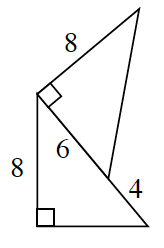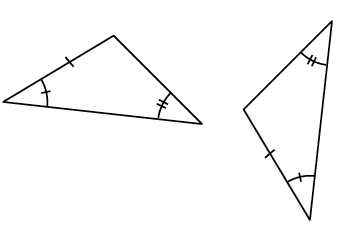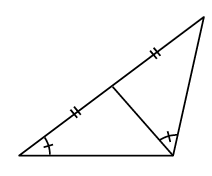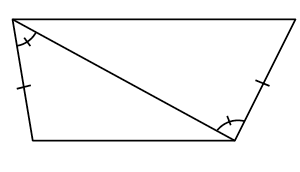### Home > GC > Chapter 6 > Lesson 6.2.4 > Problem6-71

6-71.

Determine whether or not the triangles in each pair below are congruent. Justify your conclusion.

1.Find the lengths of the missing sides using the Pythagorean Theorem.

Congruent by $\text{HL}≅$, $\text{SSS}≅$, $\text{SAS}≅$.

1.What do the tick marks on the angles and sides mean?

1.Do the tick marks prove the triangles are congruent, or is more information needed?

They are not necessarily congruent. There is no such thing as $\text{SAS}≅$.

1.Does the fact that they share a side help prove they are congruent?